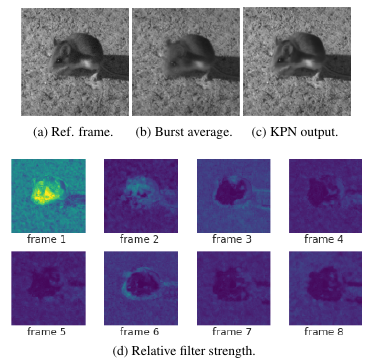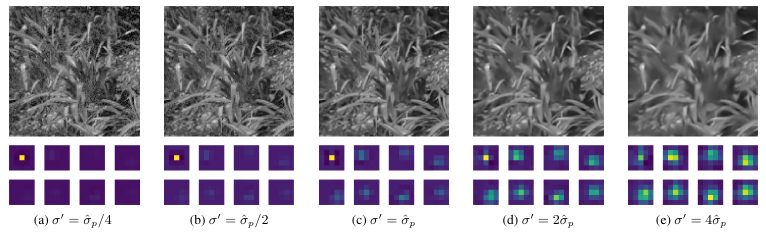# Goal

The presented approach aims at learning a set of filters for denoising bursts of images taken by hand-held cameras (e.g. in smartphones).

# Contributions

• Synthetic data creation from general purpose images simulating characteristics of real cameras.
• Pixel-wise 3D kernel prediction for denoising of burst image sequence.
• Generalisation to multiple noise levels.

# Conceptual overview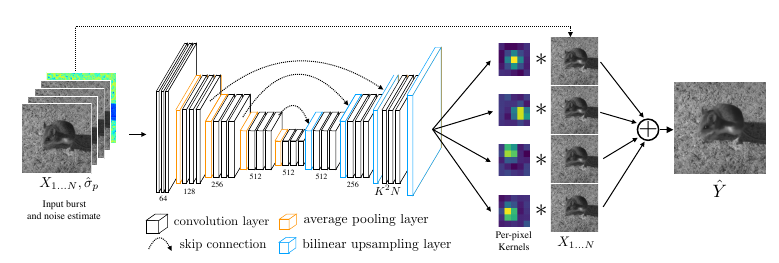#### Basic concept

• input

• set of series of N images (burst)
• one image of pixel-wise noise estimates
• encoder-decoder structure

• output

• N filters per pixel in input space (filter size: $$K$$ by $$K$$)
• synthesis of output image at pixel $$p$$ by

$\hat{Y}^p = \frac{1}{N} \sum_{i=1}^N \, <f_i^p, V^p(X_i)>,$

where $$f^p_i$$ denotes the learned filter at pixel $$p$$ in input image $$i$$.

#### Loss

• main objective

• $$L^2$$-term on gamma-corrected images
• $$L^1$$-term on gradients of gamma-corrected images

$\ell(\hat{Y}, Y^\ast) = \lambda_2 \, \left\lVert\Gamma(\hat{Y}) - \Gamma(Y^\ast)\right\rVert_2^2 + \lambda_1 \, \left\lVert\nabla\Gamma(\hat{Y}) - \nabla\Gamma(Y^\ast)\right\rVert_1^2$
• annealed loss (This is the actual loss term!)

• time dependent individual image loss term
• idea: steer training in the beginning to avoid convergence to local minima

$\mathcal{L}(X; Y^\ast, t) = \ell\left(\frac{1}{N}\sum_{i=1}^N \, f_i(X_i), Y^\ast\right) + \beta\alpha^t \sum_{i=1}^N \, \ell(f_i(X_i), Y^\ast)$

#### Synthetic data creation

• The authors develop an approach to model several artifacts involved in creation of raw data from real camera sensors, including e.g.

• explicit model for signal noise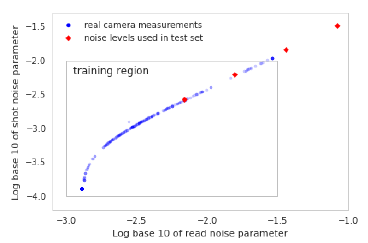• simulated misalignment due to sensor movement

# Experiments

#### Settings

• filter size: K = 5
• number of input images: N = 8

#### Nomenclature (KPN methods)

• 1-frame: N = 1
• no ann: basic loss function only (@see main objective)
• sigma blind: no noise estimate as input
• direct: directly synthesise output pixel values (by adding three additional conv layers)

#### Synthetic data set

• KPN always outperforms state of the art
• multi-frame info, annealing loss and noise estimate are all helpful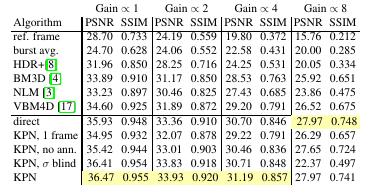#### Real data set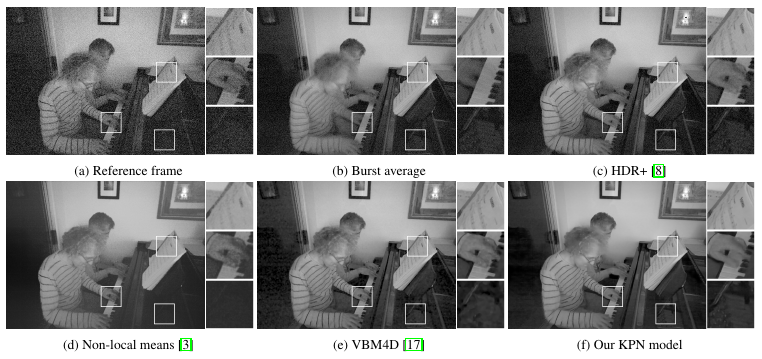#### Predicted kernels

• The approach is robust to object movement (the mouse).
• The authors claim that their ‘annealing’ approach helps focusing on a single frame where movement occurs, while taking advantage of all frames in static parts of the image (i.e. background).